maths > wholenumbers

Subtraction: First Principles

what you'll learn...

overview

This page introduced subtraction of whole numbers as taking away a part of the quantity and counting or measuring the remaining quantity.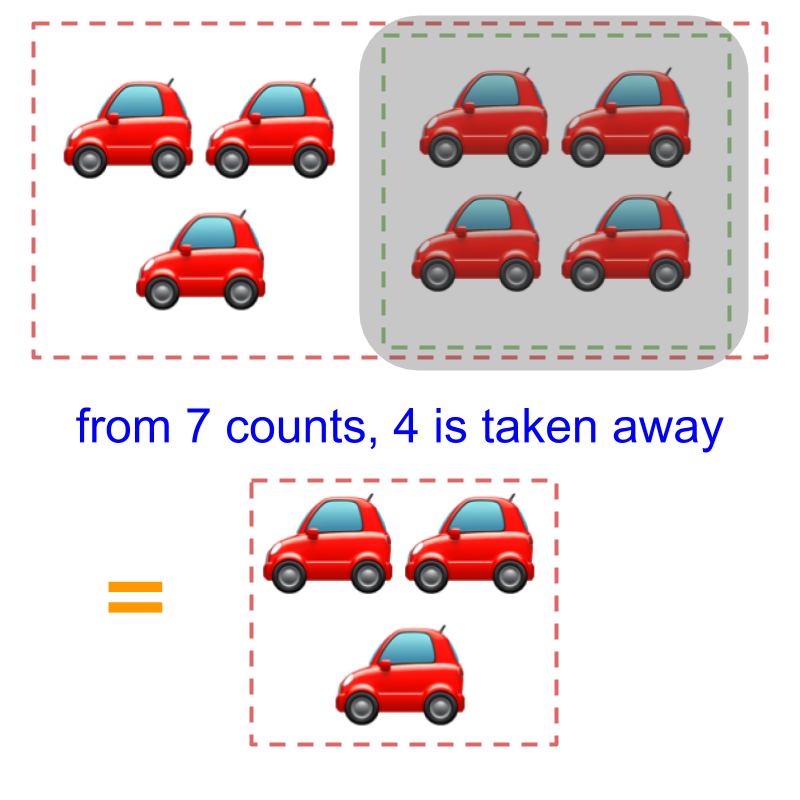4$4$ is taken away from 7$7$ and the resulting count is 3$3$.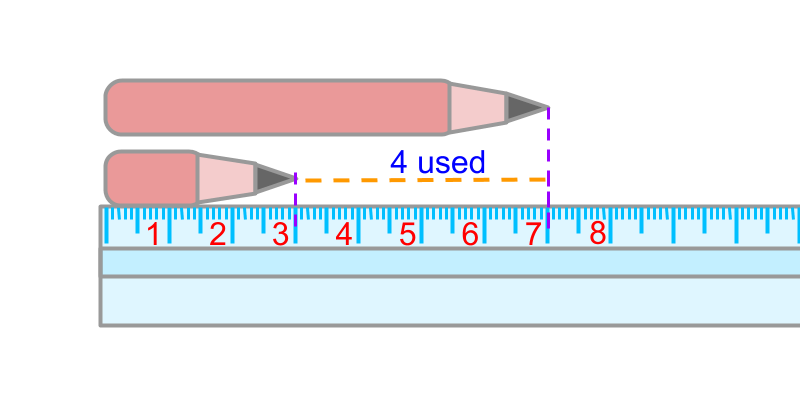The length of the pencil is 7$7$ centimeters. The length used up is 4$4$ centimeters. The remaining length is 3$3$ centimeters. The same is abstracted for subtraction in number-line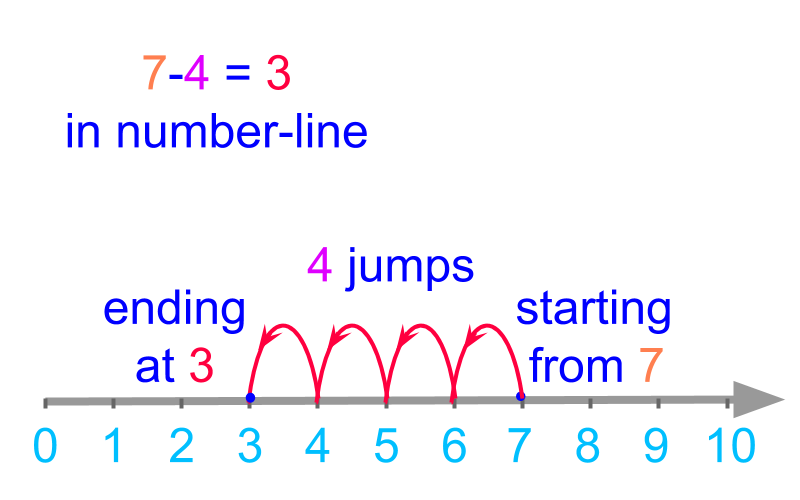The definition of subtraction in first principle forms the basis to understanding the simplified procedure for subtraction of large numbers.

take away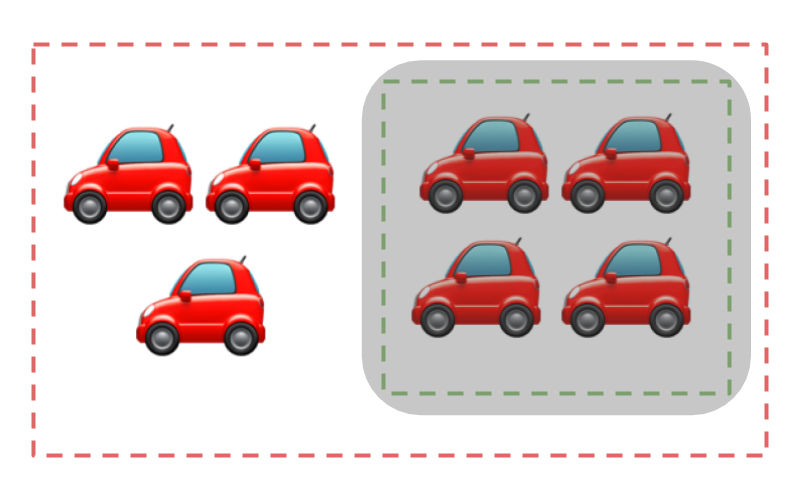There are $7$ cars shown in the figure. Out of which $4$ are taken away. The number of cars remaining is $3$.

$4$ is taken away from $7$ and the counting is carried out on the remaining cars from $1$ to $3$. The resulting count is $3$.Considering taking away $4$ cars from a group of $7$ cars.

$4$ is taken away from $7$ and the counting is carried out on the remaining cars from $1$ to $3$. The resulting count is $3$.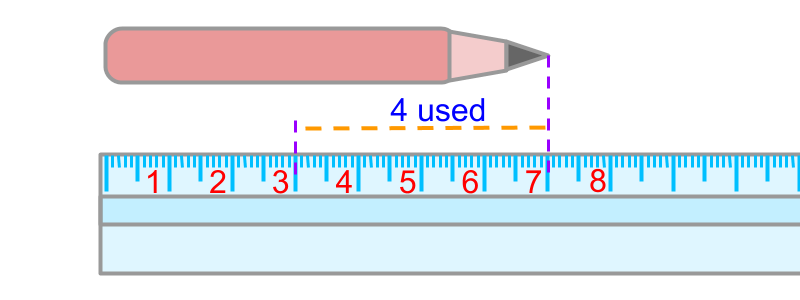A pencil is 7 centimeters in length. A person used 4 centimeters of that pencil. The length of the remaining pencil is to be found.The length of the pencil is $7$ centimeters. The length used up is $4$ centimeters.
The remaining pencil is measured and the measure at the end is $3$. The remaining length is $3$ centimeters.

A part of the count or measure of quantities can be taken-away or subtracted.

The subtraction results in count or measure of the remaining quantity.Subtraction $7 - 4$ is given in number line. The count is started from $7$ and $4$ backward-jumps are made to land in $3$. The result of the subtraction is $3$.

minuend, subtrahend, difference

The word "subtraction" means: removing part from a quantity and measuring or counting the remaining quantity.

Subtraction - First Principles : Two numbers are considered, each of which represents a count or measurement. From one amount represented by the first number, the amount represented by the second is taken away to form a result representing the remaining amount. The count or measurement of the remaining amount is the result of subtraction.

eg: $20 - 13 = 7$

$20$ is the minuend

$13$ is the subtrahend

$7$ is the difference

The minuend is, the number from which another number is subtracted.

The subtrahend is, the number that is subtracted from another number.

The difference is, the result of subtraction.

What is the difference $4 - 2$?
The answer is '$2$'.

summary

Subtraction - First Principles : Two numbers are considered, each of which represents a count or measurement. From one amount represented by the first number, the amount represented by the second is taken away to form a result representing the remaining amount. The count or measurement of the remaining amount is the result of subtraction.

eg: $20 - 13 = 7$

$20$ is the minuend

$13$ is the subtrahend

$7$ is the difference

Outline